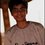# Easy but tough problem!

I just came across this question, which looks really easy to solve at the first sight, but took me hours to come up with nothing relevant. Here it is-

Given, if-

$$a^2 + b^2 - 2a - b + 10 = 0$$, Find the value of $$a + b$$.

It was a Multiple Choice Question, and hence it had options-

• $4$
• $5$
• $6$
• $8$.

Please provide with a very simple solution, as this problem doesn't seem to be the one which should be given high-level attention.Note by Akshat Jain
6 years, 10 months ago

This discussion board is a place to discuss our Daily Challenges and the math and science related to those challenges. Explanations are more than just a solution — they should explain the steps and thinking strategies that you used to obtain the solution. Comments should further the discussion of math and science.

When posting on Brilliant:

• Use the emojis to react to an explanation, whether you're congratulating a job well done , or just really confused .
• Ask specific questions about the challenge or the steps in somebody's explanation. Well-posed questions can add a lot to the discussion, but posting "I don't understand!" doesn't help anyone.
• Try to contribute something new to the discussion, whether it is an extension, generalization or other idea related to the challenge.

MarkdownAppears as
*italics* or _italics_ italics
**bold** or __bold__ bold
- bulleted- list
• bulleted
• list
1. numbered2. list
1. numbered
2. list
Note: you must add a full line of space before and after lists for them to show up correctly
paragraph 1paragraph 2

paragraph 1

paragraph 2

[example link](https://brilliant.org)example link
> This is a quote
This is a quote
    # I indented these lines
# 4 spaces, and now they show
# up as a code block.

print "hello world"
# I indented these lines
# 4 spaces, and now they show
# up as a code block.

print "hello world"
MathAppears as
Remember to wrap math in $$ ... $$ or $ ... $ to ensure proper formatting.
2 \times 3 $2 \times 3$
2^{34} $2^{34}$
a_{i-1} $a_{i-1}$
\frac{2}{3} $\frac{2}{3}$
\sqrt{2} $\sqrt{2}$
\sum_{i=1}^3 $\sum_{i=1}^3$
\sin \theta $\sin \theta$
\boxed{123} $\boxed{123}$

Sort by:

If $a$ and $b$ are complex numbers, then we can conclude straightaway that this equation will have infinitely many solutions (four obvious solutions yielding different sums: $(a,b)= (1 \pm 3i, 0)$, $(a,b)= \left (0, \frac{1\pm \sqrt{39}i}{2} \right )$). If, however, $a$ and $b$ are real, we can re-arrange the equation and obtain: $(a-1)^2 + \left (b-\frac{1}{2} \right )^2 + \frac{35}{4} = 0$ However, $(a-1)^2+\left (b-\frac{1}{2} \right )^2 \geq 0$, which leads to the conclusion $\frac{35}{4} \leq 0$, an obvious contradiction.

- 6 years, 10 months ago

Shouldn't it be $\frac{35}{4}$ instead of $\frac{17}{20}$?

- 6 years, 10 months ago

Corrected. Thanks.

- 6 years, 10 months ago

The problem seems erroneous to me. If you put a 2 behind b then the answer is 4.

- 6 years, 10 months ago

It might be possible that there is some flaw in the question. But not considering it.

- 6 years, 10 months ago

I think the options are given wrong.One can see that if we arrange the terms as:($a^{2}$ $-$ $2a$ $+$ $10$) $+$ ($b^{2}$ $-$ $b$) = $0$. Therefore, ($a^{2}$ $-$ $2a$ $+$ $10$) = $0$ and ($b^{2}$ $-$ $b$) = $0$.Now observing the first equation we see that it's having all its roots imaginary and $b$ = $0$ or $b$ = $1$ . Therefore, the result is not $0$ and is instead imaginary.Also, one can arrange the terms on L.H.S as $(a^{2}$ $-$ $2a$) $+$ ($b^{2}$ $-$ $b$ + $10$) = $0$, in this case also the same case arises an the expression is not equal to zero. One can also look as it as:($a^{2}$ $-$ $2a$) + ($b^{2}$ $-$ $b$) = $-10$ $=>$ $a(2-a)$ + $b(1-b)$ = $10$ Now, using $AM \geq GM$ , we get $1 \geq a(2-a)$ and $\frac {1}{4}\ \geq b(1-b)$.On adding we get, $\frac {5}{4}\ \geq 10$ which is a contradiction. Also,from this we find that the minimum value of the expression on the left hand side is in fact 8.75 or $\frac {35}{4}$ . Hence,for no $a$,$b$ $a$ + $b$ is equal to any of the options you have given.

- 6 years, 10 months ago

My dear, $(a^2-2a+10) + (b^2-b)=0$ doesn't imply both summands are zero. (Think of the case when one is positive & one is negative). Also don't be under the misconception that $b^2-b > 0$ for all b.(Think what happens when $b < 1$). Also $AM-GM$ applies iff all numbers you are dealing with are positive reals.

- 6 years, 10 months ago

Thanks.I get that.

- 6 years, 10 months ago

I found this question in a coaching test paper. The same exact options are given.

- 6 years, 10 months ago